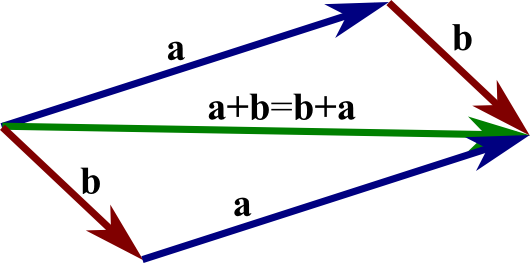# Math Insight

### Image: The parallelogram law, or commutative law, of vector additionThe parallelogram demonstrates that one obtains the same vector by adding $\vc{a}+\vc{b}$ or by adding $\vc{b}+\vc{a}$. The sum $\vc{a}+\vc{b}$ is formed by putting the tail of $\vc{b}$ at the head of $\vc{a}$ and creating the vector from the tail of $\vc{a}$ to the head of $\vc{b}$. The sum $\vc{b}+\vc{a}$ is formed by putting the tail of $\vc{b}$ at the head of $\vc{a}$ and creating the vector from the tail of $\vc{b}$ to the head of $\vc{a}$. The parallelogram shows that both of these vectors are the same diagonal of the parallelogram.

Image file: vector_parallelogram_law.png

Source image file: vector_parallelogram_law.svg
Source image type: Inkscape SVG